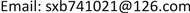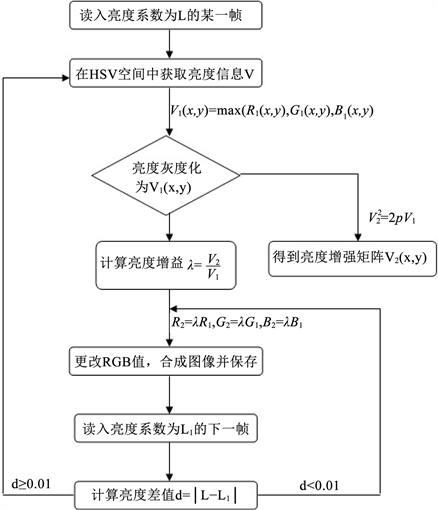﻿ 一种监控视频系统光照补偿方法 Illumination Compensation Method for Surveillance Video System

Journal of Image and Signal Processing
Vol. 08  No. 01 ( 2019 ), Article ID: 28327 , 5 pages
10.12677/JISP.2019.81004

Illumination Compensation Method for Surveillance Video System

Yong He

Technology and Information Communication Office of Zigong Public Security Bureau, Zigong SichuanReceived: Dec. 6th, 2018; accepted: Dec. 25th, 2018; published: Jan. 2nd, 2019ABSTRACT

The video illumination compensation system described in this paper can compensate the illumination of the video signal by embedding the software of the video processing system into the terminal without changing the hardware of the user’s existing video surveillance system. In this paper, the concept of brightness coefficient is defined, and the valid threshold of brightness coefficient is determined to decide whether to enter the illumination compensation module or not. In the illumination compensation algorithm, this paper calculates the difference of brightness coefficients between two frames to decide whether to use the brightness increment of the previous frame in the process of video inter-frame processing, which effectively reduces the running time of the program.

Keywords:Video Surveillance System, Illumination Compensation, Brightness Coefficient, Embedding MethodCopyright © 2019 by author and Hans Publishers Inc.1. 引言

2. 抛物线函数法亮度增强Figure 1. Flow chart of illumination compensation module

3. 视频光照补偿系统

3.1. 基本思路

$L=\frac{\underset{j=1}{\overset{255}{\sum }}i}{m\ast n}$

3.2. 算法步骤

(1) 读取当前视频帧图像 $f\left(x,y\right)$ ，其亮度系数为L；

(2) 采用HSV色度空间实现亮度和颜色分离，其中V分量为亮度信息。

(3) 在HSV空间中将图像按亮度信息灰度化，即：

${V}_{1}\left(x,y\right)=\mathrm{max}\left({R}_{1}\left(x,y\right),{G}_{1}\left(x,y\right),{B}_{1}\left(x,y\right)\right)$

(4) 获取亮度增量 $\lambda$ ：采用抛物线函数对亮度进行非线性变换，即：

${V}_{2}^{2}=2p{V}_{1}$

(5) 更改每个像素RGB值：

${R}_{2}=\lambda R{}_{1}$

${G}_{2}=\lambda {G}_{1}$

${B}_{2}=\lambda {B}_{1}$

(6) 对进入光照补偿模块的下一帧图像 ${f}_{1}\left(x,y\right)$ ，它的亮度系数为L1，求取它与前一视频帧图像 $f\left(x,y\right)$ 亮度系数的差值 $d=|L-{L}_{1}|$

(7) 如果 $d<0.01$ ，则用步骤(4)获得的 $\lambda$ 处理该视频帧图像，否则重复步骤(2)。

4. 结束语

Illumination Compensation Method for Surveillance Video System[J]. 图像与信号处理, 2019, 08(01): 24-28. https://doi.org/10.12677/JISP.2019.81004

1. 1. 肖燕峰. 基于Retinex理论的图像增强陝复算法研究[D]: [硕士学位论文]. 上海: 上海交通大学, 2007.

2. 2. Yu, Z.Y. and Bajaj, C. (2004) A Fast and Adaptive Method for Image Contrast Enhancement. Proceedings of 2004 IEEE International Conference on Image Processing, Chicage, IEEE, 2, 1001-1004.

3. 3. 梁琳, 何卫千, 雷蕾, 等. 光照不均图像增强算法综述[J]. 计算机应用研究, 2010, 27(5): 1625-1628.

4. 4. 焦竹青. 基于同态滤波的彩色图像光照补偿万法[J]. 光电子•激光, 2010, 21(4): 602-605.

5. 5. Zafar, I.M., Abdul, G. and Masood, S.A. (2013) Satellite Image Resolution Enhancement Using Dual—Tree Complex Wavelet Transformand Nonlocal Means. IEEE Geoscience and Remote Sensing Letters, 10, 451-455.

6. 6. 蒋永馨, 王孝通, 徐晓刚, 等. 一种基于光照补偿的图像增强算法[J]. 电子学报, 2009, 37(4): 151-155.

7. 7. 梁晓辉, 游志胜. 自适应的彩色图像光照补偿新方法[J]. 光电工程, 2006, 33(2): 94-97.

8. 8. 师一华. 人体肤色信息分析与检测[D]: [硕士学位论文]. 郑州: 郑州大学, 2006: 18-19.

9. 9. 杨金锋, 傅周宇, 谭铁牛, 等. 一种新型的基于内容的图像识别与过滤方法[J]. 通信学报, 2004, 25(7): 93-106.

10. 10. 蔡利梅, 钱建生, 罗驱波, 等. 一种基于色彩保持的低照度图像增强算法[J]. 计算机应用与软件, 2009, 26(3): 226-227.